# RD Sharma Solutions - Chapter 9 - Linear Equation in One Variable (Part - 1), Class 8, Maths Notes | Study RD Sharma Solutions for Class 8 Mathematics - Class 8

## Class 8: RD Sharma Solutions - Chapter 9 - Linear Equation in One Variable (Part - 1), Class 8, Maths Notes | Study RD Sharma Solutions for Class 8 Mathematics - Class 8

The document RD Sharma Solutions - Chapter 9 - Linear Equation in One Variable (Part - 1), Class 8, Maths Notes | Study RD Sharma Solutions for Class 8 Mathematics - Class 8 is a part of the Class 8 Course RD Sharma Solutions for Class 8 Mathematics.
All you need of Class 8 at this link: Class 8

PAGE NO 9.5:

Question 1:

Solve each of the following equation and also verify your solution: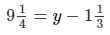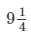= y−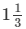or 37/4 + 4/3 = y

or y = 127/12

∴ y = 127/12 for the given equation.

Check:

L.H.S =R.H.S = 127/12−= 127/12−4/3 = (127−16)/12 = 111/12 =So, L.H.S = R.H.S for y = 127/12

PAGE NO 9.5:

Question 2:

Solve each of the following equation and also verify your solution:
5x/3 + 2/5 = 1

5x/3 + 2/5 = 1

⇒ 5x/3 = 1−2/5

⇒ 5x/3 = 3/5

⇒ x = 3/5 × 3/5 = 9/25

Verification:

L.H.S. = 5/3 × 9/25 + 2/5 = 3/5 + 2/5 = 1

R.H.S. = 1

∴ L.H.S. = R.H.S. for x = 9/25

PAGE NO 9.5:

Question 3:

Solve each of the following equation and also verify your solution:
x/2 + x/3 + x/4 = 13

x/2 + x/3 + x/4 = 13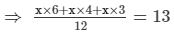⇒ 13x/12 = 13

⇒ x = 13 × 12/13 = 12

Verification:

L.H.S. = 12/2 + 12/3 + 12/4 = 6 + 4 + 3 = 13 = R.H.S.

PAGE NO 9.5:

Question 4:

Solve each of the following equation and also verify your solution:
x/2 + x/8 = 1/8

x/2 + x/8 = 1/8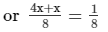or 5x/8 = 1/8

or x = 1/8 × 8/5 = 1/5

Verification:

L.H.S. = 1/2 × 1/5 + 1/8 × 1/5 = 1/10 + 1/40 = 5/40 = 1/8 = R.H.S.

PAGE NO 9.5:

Question 5:

Solve each of the following equation and also verify your solution:

2x/3−3x/8 = 7/12

2x/3−3x/8 = 7/12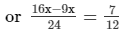or 7x/24 = 7/12

or x = 7/12 × 24/7 = 2

Verification:

L.H.S. = 4/3−6/8

=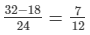R.H.S. = 7/12

∴ R.H.S. = L.H.S. for x = 2

PAGE NO 9.5:

Question 6:

Solve each of the following equation and also verify your solution:
(x  + 2)(x  + 3) + (x − 3)(x − 2) − 2x(x  + 1) = 0

(x + 2)(x + 3) + (x−3)(x−2)−2x(x + 1) = 0

or x2 + 5x + 6 + x2−5x + 6−2x2−2x = 0

or 12−2x = 0

or x = 12/2 = 6

Verification:

L.H.S. = (6 + 2)(6 + 3) + (6−3)(6−2)−2 × 6(6 + 1)

= 72 + 12−84 = 0 = R.H.S.

AGE NO 9.5:

Question 7:

Solve each of the following equation and also verify your solution:

x/2−4/5 + x/5 + 3x/10 = 1/5

x/2−4/5 + x/5 + 3x/10 = 1/5

or x/2 + x/5 + 3x/10 = 1/5 + 4/5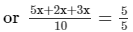or 10x/10 = 1

or x = 1

Verification:

L.H.S. = 1/2−4/5 + 1/5 + 3/10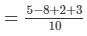= 1/5 = R.H.S.

PAGE NO 9.5:

Question 8:

Solve each of the following equation and also verify your solution:
7/x + 35 = 1/10

7/x + 35 = 1/10

or 7/x = 1/10−35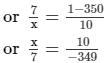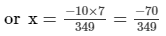Verification: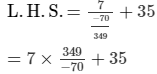= 349/−10 + 35 = 1/10 = R.H.S.

PAGE NO 9.5:

Question 9:

Solve each of the following equation and also verify your solution: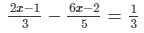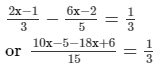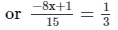or −24x + 3 = 15

or 24x = 3−15

or x = −12/24 = −1/2

Verification:

L.H.S. =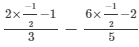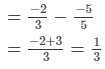PAGE NO 9.5:

Question 10:

Solve each of the following equation and also verify your solution:
13(y − 4) − 3(y − 9) − 5(y  + 4) = 0

13(y−4)−3(y−9)−5(y + 4) = 0

or 13y−52−3y + 27−5y−20 = 0

or 5y = 45

or y = 45/5 = 9

Verification:

L.H.S. = 13(9−4)−3(9−9)−5(9 + 4)

= 13 × 5−3 × 0−5 × 13

= 0 = R.H.S.

PAGE NO 9.5:

Question 11:

Solve each of the following equation and also verify your solution:
2/3(x−5)−1/4(x−2) = 9/2

2/3(x−5)−1/4(x−2) = 9/2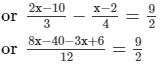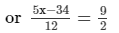or 10x−68 = 108

or 10x = 108 + 68

or x = 176/10 = 88/5

Verification:

L.H.S. = 23(885−5)−14(885−2)

= 2/3 × 63/5−1/4 × 78/5 = 9/2 = R.H.S.

The document RD Sharma Solutions - Chapter 9 - Linear Equation in One Variable (Part - 1), Class 8, Maths Notes | Study RD Sharma Solutions for Class 8 Mathematics - Class 8 is a part of the Class 8 Course RD Sharma Solutions for Class 8 Mathematics.
All you need of Class 8 at this link: Class 8Use Code STAYHOME200 and get INR 200 additional OFF

## RD Sharma Solutions for Class 8 Mathematics

88 docs

Track your progress, build streaks, highlight & save important lessons and more!

,

,

,

,

,

,

,

,

,

,

,

,

,

,

,

,

,

,

,

,

,

,

,

,

,

,

,

;